Courses

# Test: Arches -2

## 10 Questions MCQ Test Topicwise Question Bank for Civil Engineering | Test: Arches -2

Description
This mock test of Test: Arches -2 for GATE helps you for every GATE entrance exam. This contains 10 Multiple Choice Questions for GATE Test: Arches -2 (mcq) to study with solutions a complete question bank. The solved questions answers in this Test: Arches -2 quiz give you a good mix of easy questions and tough questions. GATE students definitely take this Test: Arches -2 exercise for a better result in the exam. You can find other Test: Arches -2 extra questions, long questions & short questions for GATE on EduRev as well by searching above.
QUESTION: 1

Solution:
QUESTION: 2

Solution:
QUESTION: 3

### A symmetrical circular arch of span 25 m with a central rise of 5 m is hinged at crown and springings. it carries a point load on the arch at a distance of 6.25 m from the left hinge. If the inclination of the thrust at the right hinge is 0 measured from horizontal, then what is the value of tan 0?

Solution:
QUESTION: 4

A three hinged arch is subjected to point load at crown. The magnitude of horizontal reaction at A is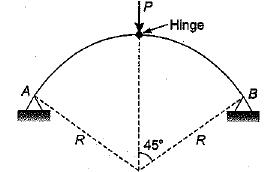Solution:
QUESTION: 5

The horizontal thrust at support A in a three hinged arch shown in the given figure is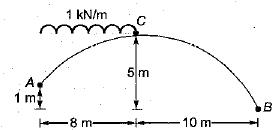Solution:
QUESTION: 6

A three hinged arch shown in the figure is quarter of a circle. If the vertical and horizontal components of reaction at A are equal, the value of θ is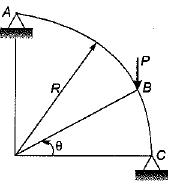Solution:
QUESTION: 7

For the semicircular two-hinged arch shown in the figure below a moment 50 t-cm applied at B produces a displacement of 0.5 cm at A. If a concentrated load of 10 t is applied at A, the rotation at B in the arch will be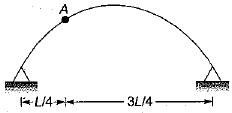Solution:
QUESTION: 8

A two hinged semicircular arch of radius 20 meters carries a load system shown in figure. The horizontal thrust at each support is (Assume uniform flexural rigidity)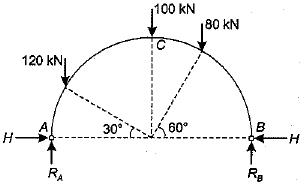Solution:
QUESTION: 9

A three hinged semicircular arch of radius R carries a uniformly distributed load w per unit run over the whole span. The horizontal thrust is

Solution:
QUESTION: 10

The horizontal thrust due to rise in temperature in a semicircular two hinged arch of radius R is proportional to

Solution: